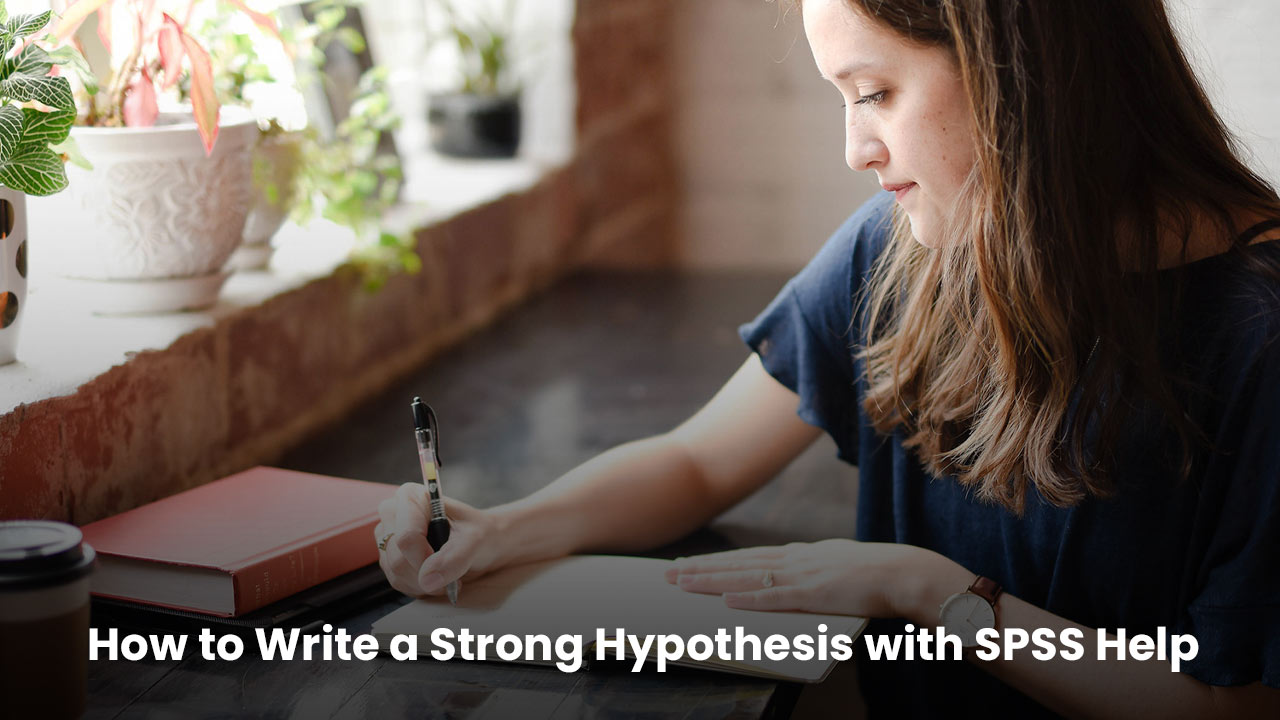# How to Write a Strong Hypothesis with SPSS Help## Introduction

When IBM developed SPSS software, its main objective was to help research scholars, especially with their research assignments such as writing research papers, hypotheses, literature reviews, dissertations, and other academic writings. This blog post is focused towards writing a strong hypothesis for your research. We will try to throw some light on writing tips for a convincing hypothesis and the role of SPSS help in it.
A hypothesis statement shows the assumptions of a researcher when he/she performs an experiment. Therefore, one must have a proper knowledge of writing a well-structured and captivating hypothesis. We can understand how difficult it is for you to construct a hypothesis and it is because you lack the knowledge of the right way to structure it perfectly. Let us help you here by sharing some wonderful writing tips and methodologies.

## What is a hypothesis?

We call a hypothesis a prediction which behaves like a tentative answer to the unproved research queries. It is not a vague guess, but an assumption of an experiment’s outcome. You start a hypothesis with a research question which is further analysed by conducting various experiments and extensive research. There might be cases where you might need to state different hypotheses for different aspects of a research question.
A hypothesis is used for decoding a research question at an initial level which is further supported by facts and pieces of evidence you have at your hand. A research problem always begins with identifying and recognising the problems first and then noting down the questions related to them. Furthermore, the initial phase of research will be conducted by performing various experiments and answering the research questions. Before performing the experiments, the outcomes are predicted based on the data and facts. This is the time when you need to make an intelligent guess. Here you write a hypothesis statement which is further proved or disproved by your study.

## What is the best way to write a hypothesis?

Now after understanding the definition of a hypothesis, let us understand how to write it.

As we mentioned earlier, a hypothesis always starts with a research question. Hence, your question must be focused and clear with the scope of research following the project limitations. Make sure the question you are going to answer has the ability to get tested and a hypothesis can be written over it.

2. ### Conduct a preliminary research

By now you must have understood how important it is to conduct initial research for a hypothesis. For your intelligent guess, gather all the necessary data that supports your answer. You can collect this data from existing theories, research papers, or studies. Using the collected data, you must draw a conclusion based on the assumption you make in answer to the question. You must be able to support your guess with some logic.

3. ### Write a hypothesis

After performing the initial research, you will get to know what more you can expect from this research and hence, here you should be precise with your answer. This information is crucial in shaping your hypothesis. This hypothesis can be expressed in different ways which depend on your project type and the structure of the hypothesis you would be using in your project.

4. ### Hypothesis refinement

A hypothesis can be refined in multiple ways. You just need to make sure that it defines all the terms clearly and includes the following statements:

1. There must be some appropriate variables.
2. Studying a specific group is necessary.
3. It is essential that your experiment or analysis includes a predicted outcome or results.
5. ### Consider three different approaches to writing your hypothesis

It is possible to phrase your predations in the form of "if...then" in order to determine the variables. As a result, you would state the independent variable at the beginning of your sentence, while the dependent variable would come at the end. During academic research, a hypothesis is presented with respect to defining the correlations or showing the effects. You need to display a direct relationship between both variables. Using the hypothesis you can tell the difference between the two variables when two groups are being compared.

6. ### Writing a null hypothesis

In the case of statistical analysis & hypothesis testing, you need to develop a null hypothesis. Use H0 as a null hypothesis and H1, and H2 as its alternatives. This null hypothesis states that there is no association between the two variables.

## Hypothesis writing tips from experts

Here we are sharing some expert tips for writing a hypothesis flawlessly.

1. Your question should be stated at the beginning.
2. It is important to understand that a hypothesis is not a question, but a statement.
3. Make sure the language you use in your hypothesis is clear and specific.
4. Remember you have to define all the variables used in your hypothesis.
5. Your hypothesis must be testable and demonstrable.
6. Analyse all the data from existing hypotheses and projects to shape your hypothesis.

## How to make your hypothesis testable

1. Think deeply about what type of experiments you will conduct to test your hypothesis.
2. Identify the variables in your project carefully.
3. Evaluate all the dependent and independent variables in your hypothesis.

##Conclusion

Writing a hypothesis is not so difficult. You just need to understand what a hypothesis is, and that’s it, all the components will start making sense to you. You just need to understand one thing, that to draft a well-written hypothesis, you should stick to the point and add testable queries. Above everything else, if you cannot write your project hypothesis by yourself for any reason, you can choose the best SPSS assignment help and services to make your hypothesis look outstanding. You will not only learn writing tactics but will also get a well-structured hypothesis. Good luck with your hypothesis!